RS Aggarwal Class 7 Solutions Chapter 15 - Properties Of Triangles

RS Aggarwal Class 7 Chapter 15 - Properties Of Triangles Solutions Free PDF

A triangle can be defined as a closed figure made of three different line segments. Triangles all have similar properties to them such as three angles and three line segments. The major properties of triangles are:

1. In a triangle, the sum of all the angles is equal to 180 degrees.
2. The sum of the lengths of any two sides of a triangle is greater than the length of the third side.
3. The longest side is always opposite to the largest angle whereas, the shortest side is opposite to the smallest angle.

The different types of triangles are categorized on the basis of the number of sides which are:

1. Equilateral triangle
2. Scalene triangle
3. Isosceles triangle

They are also categorized on the basis of angles, which are:

1. Acute angled triangle
2. Obtuse angled triangle
3. Right angled triangle

The RS Aggarwal Class 7 Solutions Chapter 15 Properties of Triangles can be found below: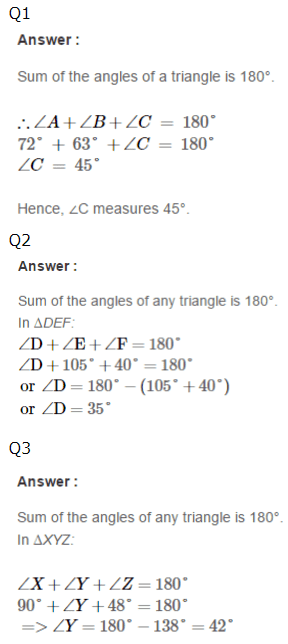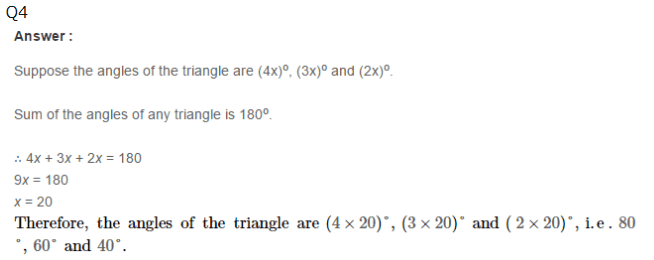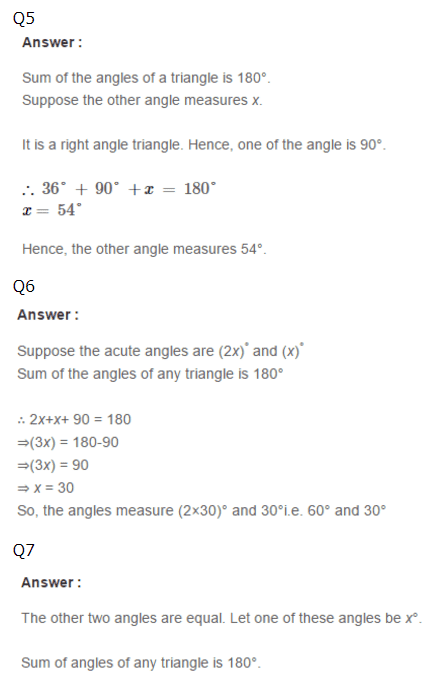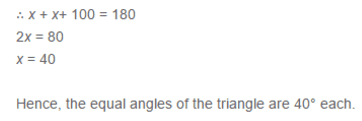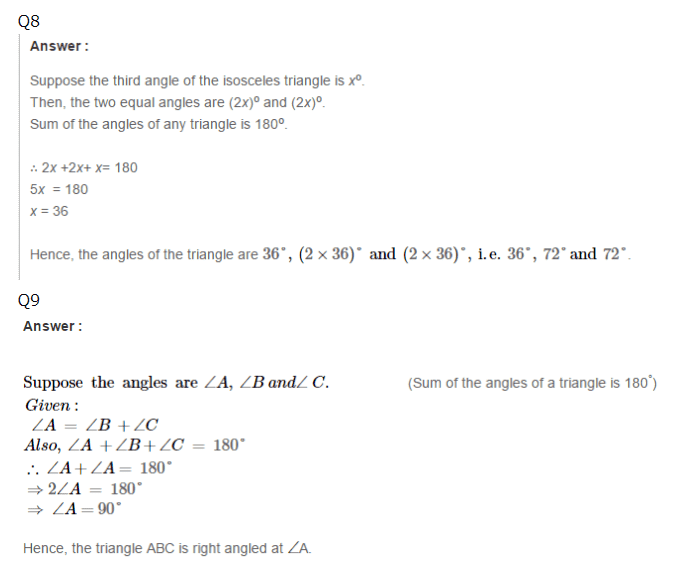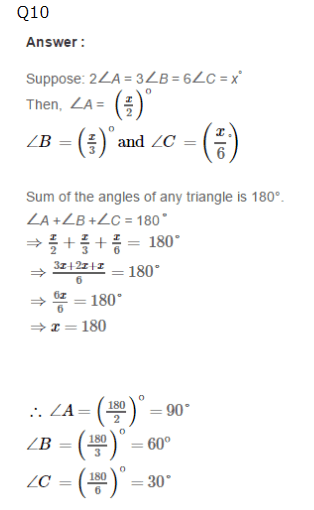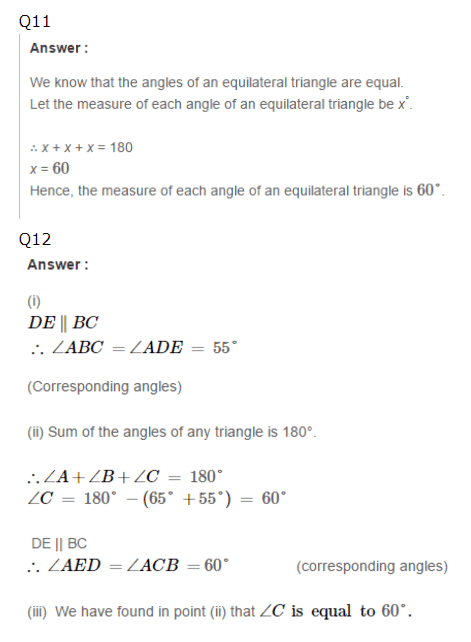Practise This Question

The correct order of the steps in the process of digestion is